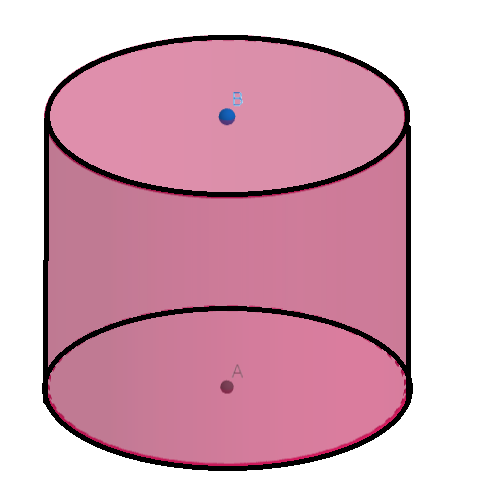QuestionAnswers

# From a sheet of area 8 square metres, a closed cylindrical tank is formed which has one-metre height and 140 cm diameter. How much area of the sheet will be left after making the tank?Hint: Assume that the area of the sheet remaining is x square centimetres. Use the fact that the curved surface area of a cylinder of radius r and height h is given by $A=2\pi rh$. Since the cylinder is closed, we need to consider both the area of the bottom and the area of the top. Use the fact that the area of a circle of radius r is equal to $\pi {{r}^{2}}$. Use the fact that the area required to make the cylinder and the area of the sheet remaining is equal to the total area of the sheet and hence form an equation in x. Solve for x and hence find the area of the sheet remaining.

Let the area of the sheet remaining be x square centimetres.We know that the curved surface area of a cylinder of radius r and height h is given by $CSA=2\pi rh$
Here the diameter of the base of the cylinder = 140cm
Hence the radius of the base of the cylinder(r) $=\dfrac{140}{2}=70cm$
Height of the cylinder(h) = 1m = 100cm
Hence the curved surface area of the cylinder $=2\pi rh=2\times \dfrac{22}{7}\times 70\times 100=44000c{{m}^{2}}$
Also, we have
Area of the top of the cylinder $=\pi {{r}^{2}}=\dfrac{22}{7}\times 70\times 70=15400c{{m}^{2}}$ and the area of the bottom of the cylinder $=\pi {{r}^{2}}=\dfrac{22}{7}\times 70\times 70=15400c{{m}^{2}}$
Hence the total area of the sheet required to make the cylinder = 44000+15400+15400=74800 square centimetres.
Also, the area of the sheet is 8 square metres = 80000 square centimetres.
Hence, we have
x+78400 = 80000
Subtracting 78400 from both sides of the equation, we get
x= 80000-78400 =1600 square centimetres
Hence the area of the sheet remaining is equal to 1600 square centimetres.
Note: In the questions of mensuration, students usually make a mistake in taking care of the units. For finding area, volume, length etc., it should be ensured that the units of all the terms included be same and or similar
Surface Area of a Prism FormulaArea of a Sector of a Circle FormulaArea of a Rhombus FormulaArea of Square FormulaCBSE Class 8 Maths Chapter 6 - Squares and Square Roots FormulasHow to Find Square Root of a NumberArea of TrapeziumTable of 8 - Multiplication Table of 8Area of Hollow CylinderArea of Triangle FormulaImportant Questions for CBSE Class 6 English Honeysuckle Chapter 8 - A Game of ChanceImportant Questions for CBSE Class 8 English Honeydew Chapter 8 - A Short Monsoon DiaryImportant Questions for CBSE Class 8 Maths Chapter 8 - Comparing QuantitiesImportant Questions for CBSE Class 6 English A Pact with The Sun Chapter 8 - A Pact with the SunImportant Questions for CBSE Class 8 English Honeydew Chapter 7 - A Visit to CambridgeImportant Questions for CBSE Class 8 Science Chapter-8 Cell - Structure and FunctionsCBSE Date SheetImportant Questions for CBSE Class 8 MathsImportant Questions for CBSE Class 8 Maths Chapter 6 - Squares and Square RootsImportant Questions for CBSE Class 8 English It So Happened Chapter 8 - JalebisCBSE Class 10 Hindi A Question Paper 2020Hindi A Class 10 CBSE Question Paper 2009Hindi A Class 10 CBSE Question Paper 2015Hindi A Class 10 CBSE Question Paper 2016Hindi A Class 10 CBSE Question Paper 2012Hindi A Class 10 CBSE Question Paper 2010Hindi A Class 10 CBSE Question Paper 2007Hindi A Class 10 CBSE Question Paper 2013Hindi A Class 10 CBSE Question Paper 2008Hindi A Class 10 CBSE Question Paper 2014RS Aggarwal Class 8 Mathematics Solutions for Chapter-18 Area of a Trapezium and a PolygonRD Sharma Solutions for Class 9 Maths Chapter 19 - Surface Area and Volume of A Right Circular CylinderRD Sharma Solutions for Class 9 Maths Chapter 20 - Surface Area and Volume of A Right Circular ConeRS Aggarwal Solutions Class 8 Chapter-18 Area of a Trapezium and a Polygon (Ex 18B) Exercise 18.2RS Aggarwal Solutions Class 8 Chapter-18 Area of a Trapezium and a Polygon (Ex 18C) Exercise 18.3RS Aggarwal Solutions Class 8 Chapter-18 Area of a Trapezium and a Polygon (Ex 18A) Exercise 18.1RD Sharma Class 8 Solutions Chapter 22 - Surface Area and Volume of Right Circular Cylinder (Ex 22.1) Exercise 22.1RD Sharma Solutions for Class 9 Maths Chapter 21 - Surface Area and Volume of a SphereRD Sharma Class 8 Solutions Chapter 22 - Surface Area and Volume of Right Circular Cylinder (Ex 22.2) Exercise 22.2RS Aggarwal Class 8 Mathematics Solutions for Chapter-20 Volume and Surface Area of Solids